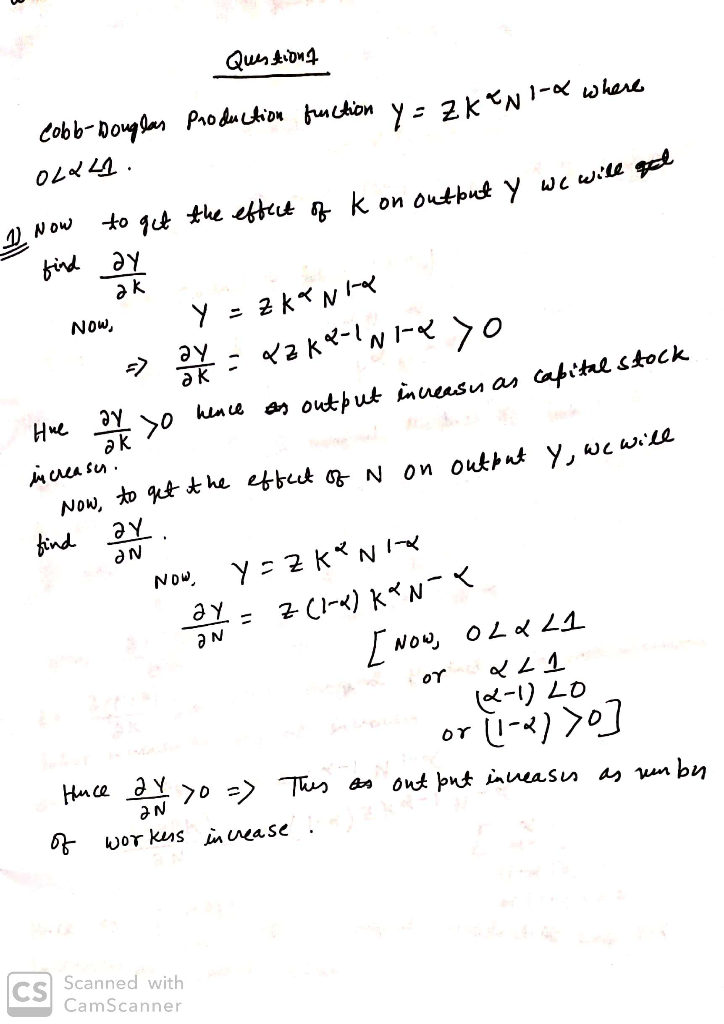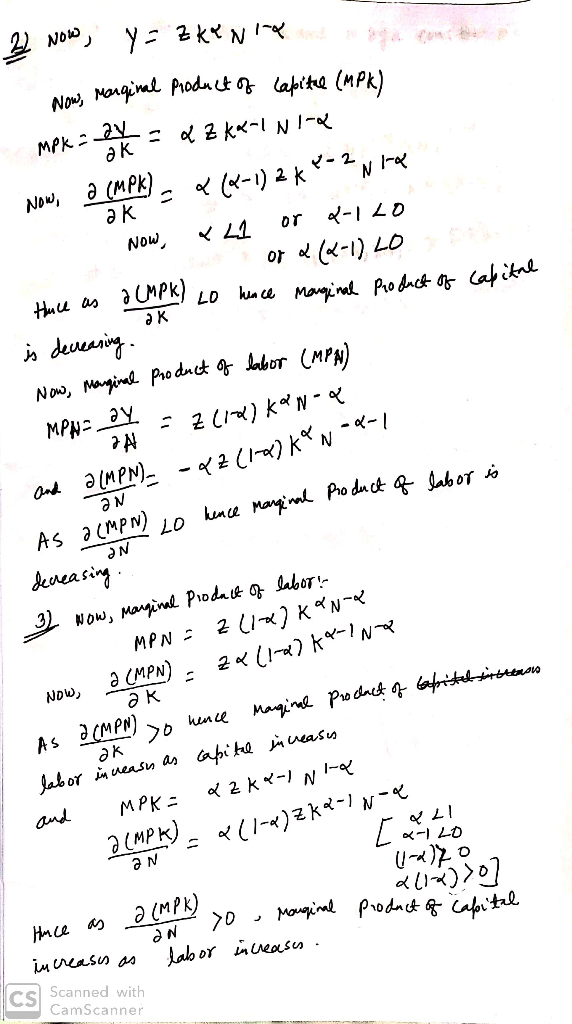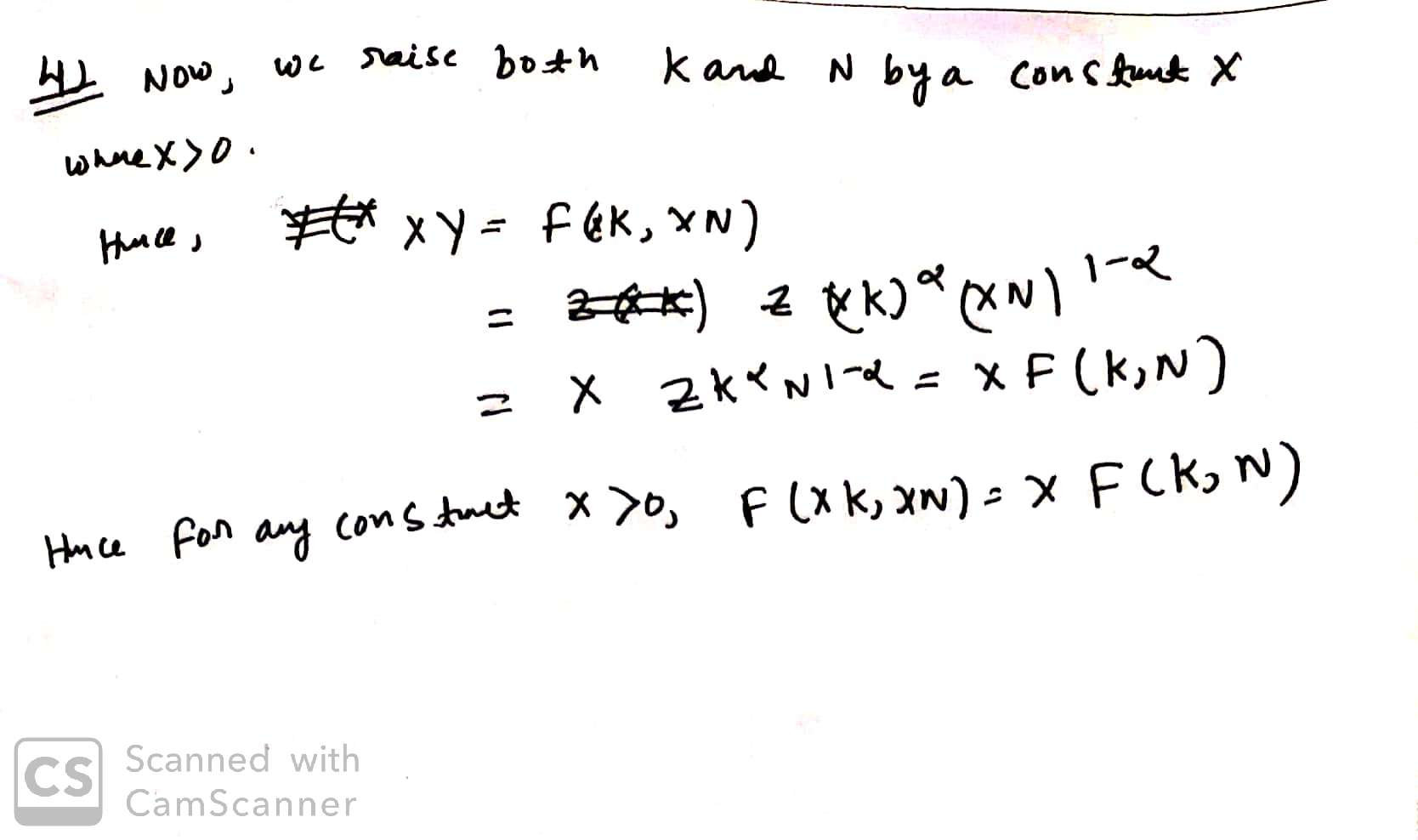In: Economics

# Question 1: Show that the Cobb-Douglas Production function Y = zKαN1−α, where 0 < α <...

Question 1: Show that the Cobb-Douglas Production function Y = zKαN1−α, where 0 < α < 1, satisfies all assumptions made in lecture 5.

Assumptions:

1) Output increases when either the capital stock or the number of workers increase

2) Both the marginal product of capital and the marginal product of labor are decreasing

3) The marginal product of labor increases when capital increases, and the marginal product of capital increases when labor increases

4) For any constant x > 0, F(xK,xN) = xF(K,N)

## Solutions

##### Expert Solution## Related Solutions

##### The total production of a particular commodity is given by the Cobb-Douglas function: f(x,y)=bx^α*y^(1−α), b is...
The total production of a particular commodity is given by the Cobb-Douglas function: f(x,y)=bx^α*y^(1−α), b is a given positive constant and 0<α<1 . Assume that we want to maximize production with a given cost constraint mx+ny−p=0 where m and n are the cost of a unit of labour and a unit of capital, respectively, and p the total cost. Show that each term will always be negative guaranteeing a maximum (in the sufficiency H test) using the values obtained of...
##### Cobb-Douglas...again Consider the Cobb-Douglas production function function of the form, q(k, l) = k α l...
Cobb-Douglas...again Consider the Cobb-Douglas production function function of the form, q(k, l) = k α l 1−α (a) Determine the relation between α and the marginal product of k and l. For what values of α is the marginal product for each input: (i) increasing, (ii) constant, and, (iii) decreasing. (b) Show that the marginal rate of technical substitution (MRTS) is equal to α 1 − α l k . For what values of α is MRTS decreasing in k?...
##### Consider the Cobb-Douglas production function ?=??^??^??^? where ?, ?, ?, ? are positive constants and ?+?+?<1....
Consider the Cobb-Douglas production function ?=??^??^??^? where ?, ?, ?, ? are positive constants and ?+?+?<1. Let ? be the amount of labor, ? the amount of capital, and ? be the amount of other materials used. Let the profit function be defined by ?=?−(??+??+??) where the costs of labor, capital, and other materials are, respectively, ?, ?, and ?. Determine whether second order conditions for profit maximization hold, when the profit function is defined by ?=?−(30?+20?+10?) with ?=5?^0.3?^0.4?^0.2.
##### An economy has a Cobb-Douglas production function: Y=K^α(LE)^1-α The economy has a capital share of 1/3,...
An economy has a Cobb-Douglas production function: Y=K^α(LE)^1-α The economy has a capital share of 1/3, a saving rate of 24 percent, a depreciation rate of 3 percent, a rate of population growth of 2 percent, and a rate of labor-augmenting technological change of 1 percent. It is in steady state. A. At what rates do total output, output per worker, and output per effective worker grow? B. Solve for capital per effective worker, output per effective worker, and the...
##### Assume a Solow model that uses Cobb-Douglas production function with and α = 0.5 for a...
Assume a Solow model that uses Cobb-Douglas production function with and α = 0.5 for a society that saves 40% of their income but sees their capital depreciate at a rate of 5%, population grows at a rate or 2% and technology grows at a rate of 3%. Determine the steady state level of capital per worker. Now assume that the economy experiences population growth of 5%, what would their savings rate need to be to make sure that the...
##### Stanford airline's production function is given by a Cobb-Douglas form: where: Y = number of passengers...
Stanford airline's production function is given by a Cobb-Douglas form: where: Y = number of passengers carried per year L = number of pilots (labor) K = number of aircraft (capital) a) show that the product elasticity for labor is given by EYL = a, and that the product elasticity for capital is given by /•'. ,.=/?. b) show that MPL > 0, MPK >0, and that tfY / âl: < 0 , &Y / cK2 < 0. c) show...
##### The production of a manufacturer is given by the Cobb-Douglas production function f(x,y)=30x^(4/5)y^(1/5) where x represents...
The production of a manufacturer is given by the Cobb-Douglas production function f(x,y)=30x^(4/5)y^(1/5) where x represents the number of units of labor (in hours) and y represents the number of units of capital (in dollars) invested. Labor costs $10 per hour and there are 8 hours in a working day, and 250 working days in a year. The manufacturer has allocated$4,000,000 this year for labor and capital. How should the money be allocated to labor and capital to maximize...
##### 1.A production function such as Y=AKaLb is called the Cobb-Douglas (C-D) production function. Only two factors...
1.A production function such as Y=AKaLb is called the Cobb-Douglas (C-D) production function. Only two factors of production are assumed here: capital (K) and labor (L), with A interpreted as the level of technology. Later, it was discovered that human capital (H) is also a "neglected"factor of production, assuming that the function satisfies constant return to scales for all the factors of production that it should include (except for technology). In the correct production function, there should be: A. a+b=1。...
##### QUESTION Consider the following Cobb Douglas production function: Y= K2/5L3/5. The rate of depreciation in the...
QUESTION Consider the following Cobb Douglas production function: Y= K2/5L3/5. The rate of depreciation in the economy is 2% and the marginal propensity to save (mps) is 30%. Any output that is not saved is consumed and this is a closed economy. Population growth rate is zero. Continue with the same data with the exception that mps is unknown. Solve for the rate of investment which will ensure golden rule of consumption per capita . Show all the steps covered...
##### Suppose a firm has a Cobb-Douglas production function. Show graphically that an increase in the rental...
Suppose a firm has a Cobb-Douglas production function. Show graphically that an increase in the rental rate of capital will increase the amount of labor hired if production remains at the same amount(10pts).